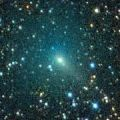# \$B%\%"%C%F%#!<%KWB@1(B

C/2008 J1 ( Boattini )###\$B%W%m%U%#!<%k(B

 \$BH/8+F|(B 2008\$BG/(B5\$B7n(B2\$BF|(B \$BH/8+8wEY(B 14.2\$BEy(B \$BH/8+ A. Boattini (Catalina Sky Survey)

###\$B###\$B50F;MWAG(B

```The following orbital elements are taken from MPC 65054:

Epoch 2008 Aug. 2.0 TT = JDT 2454680.5
T 2008 July 13.2614 TT                                  MPC
q   1.724295             (2000.0)            P               Q
z  +0.006022       Peri.   68.1226      +0.4602495      +0.1205294
+/-0.000001       Node   273.4197      -0.6425054      +0.7289323
e   0.989617       Incl.   61.7800      +0.6126640      +0.6738920
From 1521 observations 2008 May 2-2009 Jan. 22, mean residual 0".5.
```

###\$B@1?^(B###\$B8wEYJQ2=(B

```        m1 = 2.2 + 5 log\$B&\$(B + 30   log r(t - 15)  [   ,190]  (              \$B!A(B2009\$BG/(B 1\$B7n(B19\$BF|(B)
m1 = 8.5 + 5 log\$B&\$(B + 15.0 log r          [190,   ]  (2009\$BG/(B 1\$B7n(B19\$BF|!A(B              )
```##### \$B50F;MWAG\$O(BMPC\$B\$N(BEphemerides and Orbital Elements\$B\$K7G:\\$5\$l\$?\$b\$N\$G\$9!#(B \$B@1?^\$O%9%F%i%J%S%2!<%?(B Ver.8 (\$B%"%9%H%m%"!<%D(B) \$B\$G:n@.\$7\$?\$b\$N\$G\$9!#(B \$B8wEY%0%i%U\$O(BComet for Windows\$B\$G:n@.\$7\$?\$b\$N\$G\$9!#(B